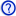# Dqo transformation

﻿
Dqo transformationAdditional information needed In electrical engineering, direct–quadrature–zero (or dq0 or dqo) transformation or zero–direct–quadrature (or 0dq or odq) transformation is a mathematical transformation used to simplify the analysis of three-phase circuits. In the case of balanced three-phase circuits, application of the dqo transform reduces the three AC quantities to two DC quantities. Simplified calculations can then be carried out on these imaginary DC quantities before performing the inverse transform to recover the actual three-phase AC results. It is often used in order to simplify the analysis of three-phase synchronous machines or to simplify calculations for the control of three-phase inverters. The dqo transform presented here is exceedingly similar to the transform first proposed in 1929 by R.H. Park. In fact, the dqo transform is often referred to as Park’s transformation.

## Definition

The dqo transform applied to three-phase currents is shown below in matrix form:$I_{dqo} = TI_{abc} = \sqrt{\frac{2}{3}}\begin{bmatrix} \cos(\theta)&\cos(\theta - \frac{2\pi}{3})&\cos(\theta + \frac{2\pi}{3}) \\ \sin(\theta)&\sin(\theta - \frac{2\pi}{3})&\sin(\theta + \frac{2\pi}{3}) \\ \frac{\sqrt{2}}{2}&\frac{\sqrt{2}}{2}&\frac{\sqrt{2}}{2} \end{bmatrix}\begin{bmatrix}I_a\\I_b\\I_c\end{bmatrix}$

The inverse transform is:$I_{abc} = T^{-1}I_{dqo} = \sqrt{\frac{2}{3}}\begin{bmatrix}\cos(\theta)&\sin(\theta)&\frac{\sqrt{2}}{2}\\ \cos(\theta - \frac{2\pi}{3})&\sin(\theta - \frac{2\pi}{3})&\frac{\sqrt{2}}{2}\\ \cos(\theta + \frac{2\pi}{3})&\sin(\theta + \frac{2\pi}{3})&\frac{\sqrt{2}}{2}\end{bmatrix} \begin{bmatrix}I_d\\I_q\\I_o\end{bmatrix}$

### Geometric Interpretation

The dqo transformation can be thought of in geometric terms as the projection of the three separate sinusoidal phase quantities onto two axes rotating with the same angular velocity as the sinusoidal phase quantities. The two axes are called the direct, or d, axis and the quadrature, or q, axis. Not surprisingly the q-axis is at an angle of 90 degrees to (in quadrature with) the direct axis.Shown above is the dqo transform as applied to the stator of a synchronous machine. There are three windings separated by 120 physical degrees. The three phase currents are equal in magnitude and are separated from one another by 120 electrical degrees. The three phase currents lag their corresponding phase voltages by δ. The d-q axis is shown rotating with angular velocity equal to ω, the same angular velocity as the phase voltages and currents. The d axis makes an angle θ = ωt with the A winding which has been chosen as the reference.The currents Id and Iq are constant DC quantities.

## Comparison With Other Transforms

### Park's Transformation

The transformation originally proposed by Park differs slightly from the one given above. Park's transformation is:$P= \frac{2}{3}\begin{bmatrix} \cos(\theta)&\cos(\theta - \frac{2\pi}{3})&\cos(\theta + \frac{2\pi}{3}) \\ \sin(\theta)&\sin(\theta - \frac{2\pi}{3})&\sin(\theta + \frac{2\pi}{3}) \\ \frac{1}{2}&\frac{1}{2}&\frac{1}{2} \end{bmatrix}$

and$P^{-1} = \begin{bmatrix}\cos(\theta)&\sin(\theta)&1\\ \cos(\theta - \frac{2\pi}{3})&\sin(\theta - \frac{2\pi}{3})&1\\ \cos(\theta + \frac{2\pi}{3})&\sin(\theta + \frac{2\pi}{3})&1\end{bmatrix}$

Although useful, Park's transformation is not power invariant whereas the dqo transformation defined above is[citation needed]. Park's transformation gives the same zero component as the method of symmetrical components. The dqo transform shown above gives a zero component which is larger than that of Park or symmetrical components by a factor of$\sqrt{3}$.

### αβγ transform

The dqo transform is conceptually similar to the αβγ transform. Whereas the dqo transform is the projection of the phase quantities onto a rotating two-axis reference frame, the αβγ transform can be thought of as the projection of the phase quantities onto a stationary two-axis reference frame.

Wikimedia Foundation. 2010.

### Look at other dictionaries:

• Alpha–beta transformation — In electrical engineering, the αβγ transformation (also known as the Clarke transformation) is a mathematical transformation employed to simplify the analysis of three phase circuits. Conceptually it is similar to the dqo transformation. One very …   Wikipedia